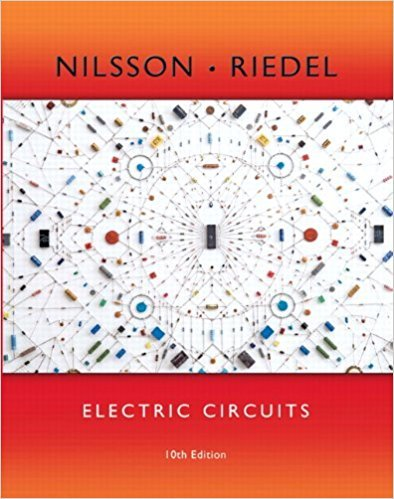×
×

# a) Determine the number of mesh-current equations needed to solve the circuit shown. b)ISBN: 9780133760033 390

## Solution for problem 4.8 Chapter 4.6

Electric Circuits | 10th Edition

• Textbook Solutions
• 2901 Step-by-step solutions solved by professors and subject experts
• Get 24/7 help from StudySoup virtual teaching assistantsElectric Circuits | 10th Edition

4 5 1 397 Reviews
10
4
Problem 4.8

a) Determine the number of mesh-current equations needed to solve the circuit shown. b) Use the mesh-current method to find how much power is being delivered to the dependent voltage source. 14 2 25 V 1 Answer: (a) 3;(b)-36 W.

Step-by-Step Solution:
Step 1 of 3

BIT 323 CAT-DATA ANALYSIS TECHNIQUES SIT/0006/10 Assignment 1 Types of Hypotheses There are two types of hypotheses: i. Research hypothesis ii. Null hypothesis. Research hypothesis It is the hypothesis derived from the researcher’s theory about some social phenomenon. Researchers always believe that their research hypotheses are accurate statements about the condition...

Step 2 of 3

Step 3 of 3

##### ISBN: 9780133760033

Electric Circuits was written by and is associated to the ISBN: 9780133760033. This textbook survival guide was created for the textbook: Electric Circuits, edition: 10. Since the solution to 4.8 from 4.6 chapter was answered, more than 213 students have viewed the full step-by-step answer. The full step-by-step solution to problem: 4.8 from chapter: 4.6 was answered by , our top Engineering and Tech solution expert on 03/13/18, 07:48PM. This full solution covers the following key subjects: . This expansive textbook survival guide covers 107 chapters, and 1357 solutions. The answer to “a) Determine the number of mesh-current equations needed to solve the circuit shown. b) Use the mesh-current method to find how much power is being delivered to the dependent voltage source. 14 2 25 V 1 Answer: (a) 3;(b)-36 W.” is broken down into a number of easy to follow steps, and 40 words.

Unlock Textbook Solution